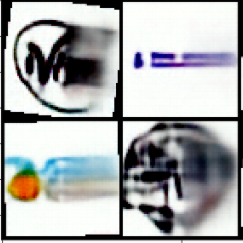# Autoencoder with skip connections, right side of the output is blurry

I created an autoencoder with skip connections whose blocks are as follows;

``````class ResidualDecoderDoublingBlock(nn.Module):
def __init__(self,in_channels,out_channels):
super().__init__()
self.in_channels, self.out_channels = in_channels,out_channels
self.block = nn.Sequential(
convT2x2(self.in_channels,self.out_channels,stride=1),
nn.BatchNorm2d(self.out_channels,eps=1e-05, momentum=0.1, affine=True),
nn.PReLU(self.out_channels),

nn.BatchNorm2d(self.out_channels,eps=1e-05, momentum=0.1, affine=True),
)
self.shortcut = nn.Sequential(
convT2x2(self.in_channels,self.out_channels,stride=2),
nn.BatchNorm2d(self.out_channels,eps=1e-05, momentum=0.1, affine=True),
)

self.activate = nn.PReLU(self.out_channels)

def forward(self,x):
residual = self.shortcut(x)
x = self.block(x)
x += residual
return self.activate(x)

class ResidualEncoderHalvingBlock(nn.Module):
def __init__(self,in_channels,out_channels):
super().__init__()
self.in_channels, self.out_channels = in_channels,out_channels
self.block = nn.Sequential(
conv2x2(self.in_channels,self.out_channels,stride=1),
nn.BatchNorm2d(self.out_channels,eps=1e-05, momentum=0.1, affine=True),
nn.PReLU(self.out_channels),

nn.BatchNorm2d(self.out_channels,eps=1e-05, momentum=0.1, affine=True),

)
self.shortcut = nn.Sequential(
conv2x2(self.in_channels,self.out_channels,stride=2),
nn.BatchNorm2d(self.out_channels,eps=1e-05, momentum=0.1, affine=True),
)

self.activate = nn.PReLU(self.out_channels)

def forward(self,x):
residual = self.shortcut(x)
x = self.block(x)
x += residual
return self.activate(x)
``````

where conv2x2 is Conv2d(kernel_size=2,bias=False), convT2x2 is ConvTranspose2d(kernel_size =2,bias=False)

I train the model I made via chaining these blocks about 25000 iterations each of which has minibatch size of 64, the latent size is [2048,1,1] per image, and loss is MSE (original pytorch implementation). I use adam optimizer with learning rate of 0.001. if my training set is small (~18.000 samples), my model overfits and I get crisp images which is fine for now. However if my training set is large (~260.000 samples) after 90.000 iterations (not epochs), the output is as follows;

the input ;the output;This is true for every output image. left side is either crisp, or blurred negligibly but the right side is blurred too much (as in unrecognizable or too much information loss), the reconstruction loss does not decrease after about 60.000 iterations. Decreasing learning rate 10 fold did not help.

I don’t know if this is due to an inherit design flaw of mine which shows up when the dataset is big, or something else.

Any solutions, or theories as to why?

My best guess would be that (some of) the newly added images might have something “special” on the right hand side. Did you check the images beforehand or verify them somehow or could it be the case, e.g. that some of these images are completely black or white on the right side etc.?

I have to check that because I did not clean the dataset (other than automatically eliminating a subset of it). If that is the case, it did not get my attention while working on it. However there could be a subset of images where your suggestion could be true. Thanks.

Edit after checking;
@ptrblck The images in my dataset dont have a subset that have a special right side, exactly. However my dataset is comprised of special kind of images (logos or logotypes in general). Most of the images are symmetric or “almost” symmetric with small changes along the vertical axis. And most of the variation is on the left side (since most interesting figures of logos are on the left side or above of text by tradition as in “sunny escape” logo shown above). My network may be learning to cheat a little by smudging a low resolution version of the leftside to the right side. Whatever the reason is, I will come back here let you guys know, after I figure out a way to solve this problem. Other ideas are also
welcome.

Thanks for the update. If you think that the sides might be “different” in any sense, could you add a random flip transformation to the training, which should hopefully get rid of these artifacts.
Let me know, if that helps.